B-Phi-structure

Letbe a fibration and letbe an-dimensional vector bundle over a space, classified by the mapping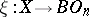. Then the homotopy class lifting the mappingto a mapping inis called a-structure on, i.e. it is an equivalence class of mappingssuch that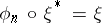, where two mappingsand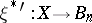are said to be equivalent if they are fibrewise homotopic. No method of consistently defining-structures for equivalent fibrations exists, because this consistency depends on the choice of the equivalence.
Let there be a sequence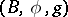of fibrations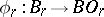and mappingssuch that(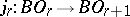is the standard mapping). The family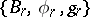(and sometimes only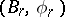) is called a structure series. An equivalence class of sequences of-structures on the normal bundle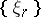of a manifold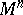is called a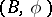-structure on; they coincide beginning from some sufficiently large. A manifoldwith a fixed-structure on it is called a-manifold.
Instead of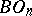, a more general space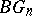, classifying sphere bundles, can be considered and-structures can be introduced on them.